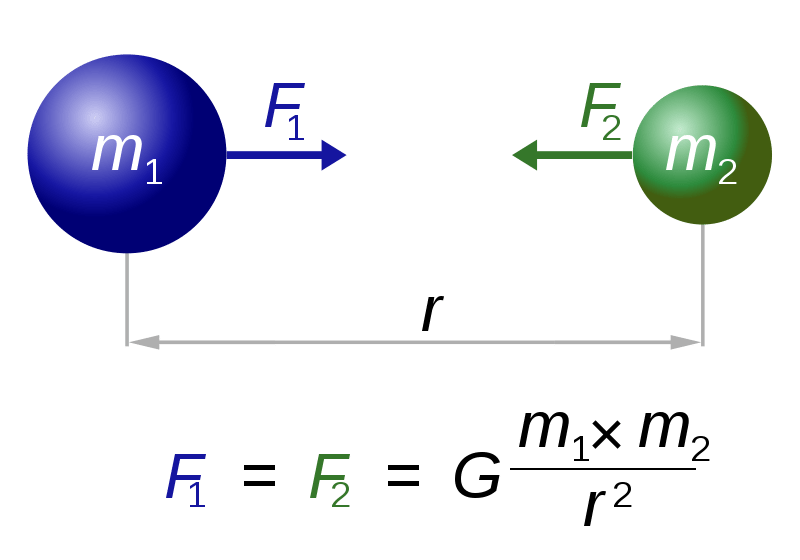# Newton’s Universal Law of Gravitation

23.06.2015

Widely known as the man who discovered gravity, 18th-century scientist Sir Isaac Newton additionally made multiple important observations about gravity’s behaviors. He formulated the first laws about gravitational physics, perhaps the most important and well-known of which is his universal law of gravitation. This law states that two objects exert gravitational force on each other, directly proportional to their masses and inversely proportional to the square of the distance that separates them.On Earth, it is easy for Newton and other physicists to observe how the large mass of the planet exerts gravitational force on all objects—giving them the concept commonly called “weight.” In fact, “weight” is really a term for how much gravity the Earth exerts on that object. It would have been easy for Newton to postulate that only large, planetary bodies exert gravitational force. The reason Newton’s universal law of gravitation is revolutionary is that he asserted, and many have proven, how gravitational force is universally exerted by all objects with mass, regardless of size or location.

Newton’s universal law of gravitation and its corresponding mathematical equation demonstrate how all mass has gravitational pull to all other mass, but also that pull is diminished by distance. Two large bodies, as they approach one another, will be affected by the gravitational pull of one another. Accordingly, the larger (the object with more mass) will exert greater gravity over the smaller and be less affected by its gravity in return. As the objects move away from each other, the force exerted on each by the other decreases.

Not only has this law been proven true for massive bodies like the planets Jupiter and Saturn, which affect each other’s orbits with their gravity, it remains true with small masses. Newton’s universal law of gravitation provided the basis of many studies in astrophysics and quantum mechanics.

Learn Something New Every Day

Get smarter with 10-day courses delivered in easy-to-digest emails every morning. Join over 400,000 lifelong learners today!